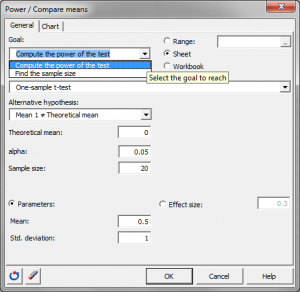# Statistical Power for mean comparison

Ensure optimal power or sample size using power analysis. Power for the comparison of means available in Excel using the XLSTAT statistical software.## Statistical Power analysis for the comparison of means

There are several tests to compare means, in XLSTAT we offer namely the t and z tests. XLSTAT allows estimating the power of these tests and calculates the number of observations required to obtain sufficient power. Power computations can also be applied for the Wilcoxon signed rank test and the Mann-Whitney test.

When testing a hypothesis using a statistical test, there are several decisions to take:

• The null hypothesis H0 and the alternative hypothesis Ha.
• The statistical test to use.
• The type I error also known as alpha. It occurs when one rejects the null hypothesis when it is true. It is set a priori for each test and is 5%.

The type II error or beta is less studied but is of great importance. In fact, it represents the probability that one does not reject the null hypothesis when it is false. We cannot fix it upfront, but based on other parameters of the model we can try to minimize it. The power of a test is calculated as 1-beta and represents the probability that we reject the null hypothesis when it is false. We therefore wish to maximize the power of the test. XLSTAT allows to calculate the power (and beta) when other parameters are known. For a given power, it also allows to calculate the sample size that is necessary to reach that power.

The statistical power calculations are usually done before the experiment is conducted. The main application of power calculations is to estimate the number of observations necessary to properly conduct an experiment. XLSTAT allows to compare:

We use the t-test when the variance of the population is estimated and the z-test when it is known. In each case, the parameters will be different and will be shown in the dialog box.

## How is Statistical Power calculated

The power of a test is usually obtained by using the associated non-central distribution. Thus, for the t-test, the non-central Student distribution is used and the z-test the Normal distribution.

### Satistical Power for a T-test or Z-test for one sample

The power of this test is obtained using the non-central Student distribution with non-centrality parameter (NCP): NCP = |(X - X0)/SD) - √N|

With X0 theoretical mean and SD standard deviation. The part X - X0)/SD is called the effect size.

### T-test or Z-test for two paired samples

The same formula as for the one sample case applies, but the standard deviation is calculated differently, we have: NCP = |(X1 - X2)/SDDiff) - √N|

With SDDiff= √(SD1² + SD2²) – 2 corr*SD1SD2 and Corr is the correlation between the two samples. The part X1 - X2)/SDDiff is the effect size.

### T-test or Z-test for two independent samples

In the case of two independent samples, the standard deviation is calculated differently and we use the harmonic mean of the number of observations.

Calculating sample size using the statistical power of a test

To calculate the number of observations required, XLSTAT uses an algorithm that searches for the root of a function. It is called the Van Wijngaarden-Dekker-Brent algorithm (Brent, 1973). This algorithm is adapted to the case where the derivatives of the function are not known. It tries to find the root of:

power (N) - expected_power

We then obtain the size N such that the test has a power as close as possible to the desired power.

## Effect size in power calculation

This concept, developed by Cohen (1988) is very important in power calculations. The effect size is a quantity that will allow calculating the power of a test without entering any parameters but will tell if the effect to be tested is weak or strong.

In the context of comparisons of means, the conventions of magnitude of the effect size are:

• d=0.2, the effect is small.
• d=0.5, the effect is moderate.
• d=0.8, the effect is strong.

XLSTAT allows entering the effect size directly.### analyze your data with xlstat

14-day free trial

Included in

Related features